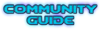## Random2() and SetRandomSeed how it works exactly?

Moderator: GZDoom Developers

Forum rules
Before asking on how to use a ZDoom feature, read the ZDoom wiki first. If you still don't understand how to use a feature, then ask here.

### Random2() and SetRandomSeed how it works exactly?

Subj.

Random2 is basically just random() - random(), i.e. it subtracts a random byte value from another random byte value.

It effectively creates a random number between -255 and 255 with the central values more likely.
random2(x) is basically random(x)-random(x). The default value for random2() call is 255, so you can get a result between -255 and +255.
Well, I've thoughts that random2(9) would returns random(0,9)-random(0,9), i. e. random(0,9) = randompick(0,1,2,3,4,5,6,7,8,9), but wiki says that random(0,9) returns randompick(0,1,8,9):
Returns a random integer value between -mask and +mask. It is roughly equivalent to random(0, mask) - random(0, mask). If no mask is provided (random2()), the maximum value of 255 is used instead. Mask is used as a binary mask, e.g. if 9 is used, the random results can be [0, 1, 8, 9] - [0, 1, 8, 9]

So, is have a mistake somewhere or I just understand something wrong about random() calculating and randomization mechanics in general? If it haven't too hard for you, can you explain it on simple examples also, for ex. which result would be in the next examples?:
random(0,9) =
random(9) =
random2(9) =
------------------------
Btw, what SetRandomSeed[identifier](seedvalue) is and for what it supposed to be used? I've heard a somewhere that it's a something for "saving" randomization result in save file. Anyway I'd wish have see at a some example of usage.Void Weaver
Keeper of the Diablo bestiary storage Also amateur DECORATE-spaghetti weaver ಠ_ಠ

Joined: 18 Dec 2014

### Re: Random2() and SetRandomSeed how it works exactly?

Void Weaver wrote: but wiki says that random(0,9) returns randompick(0,1,8,9):

Random2 is not what you may think - it's (random() & mask) - (random() & mask). So, Random2(9) is not random(0,9) - random(0,9) but effectively randompick(0,1,8,9) - randompick(0,1,8,9). Better not use the mask parameter ever, it's an old cruft from Heretic that has little use.Graf ZahlJoined: 19 Jul 2003
Location: Germany

### Re: Random2() and SetRandomSeed how it works exactly?

Oh, got it, thank you.Just another yet a bit wrong wiki description.Void Weaver
Keeper of the Diablo bestiary storage Also amateur DECORATE-spaghetti weaver ಠ_ಠ

Joined: 18 Dec 2014

### Re: Random2() and SetRandomSeed how it works exactly?

SetRandomSeed is if you need a guaranteed sequence of 'random' numbers. When you set a specific number as seed the RNG will always produce the same numbers. Normally you won't need it but there are scenarios where you may want to use an RNG for purposes where total randomness is not wanted.Graf ZahlJoined: 19 Jul 2003
Location: Germany

### Re: Random2() and SetRandomSeed how it works exactly?

So it can be used as part of anti-savescumming mechanics, ja?
For ex. if we set a row of numbers SetRandomSeed[LootChestGenerated](2,31,67,5,121), then it can return (but how?!) a same set of values for a some script or event, right?Void Weaver
Keeper of the Diablo bestiary storage Also amateur DECORATE-spaghetti weaver ಠ_ಠ

Joined: 18 Dec 2014